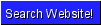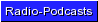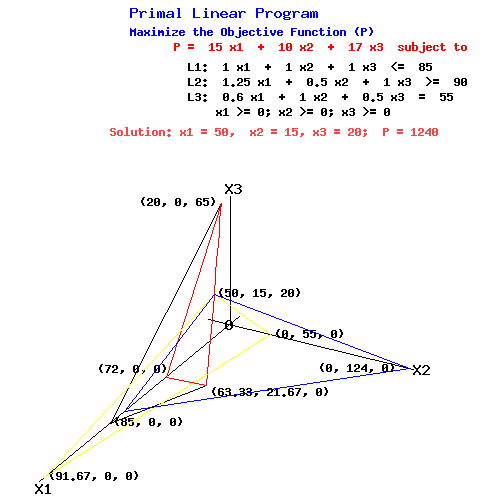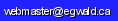Operations Research - Linear Programming - Dual Simplex Tableaux Generator

by

Elmer G. Wiens

Egwald's popular web pages are provided without cost to users.
Please show your support by joining Egwald Web Services as a Facebook Fan:Follow Elmer Wiens on Twitter:The dual simplex method transforms an initial tableau into a final tableau containing the solutions to the primal and dual problems. Each stage of the algorithm generates an intermediate tableau as the algorithm gropes towards a solution.

This web page permits you to enter your own linear programming problem and generate these tableaux with a user friendly interface. You can specify up to 6 variables and 10 constraints in the primal problem, with any mixture of <=, >=, and = constraints.

To illustrate the procedure you need to follow, consider the problem:

 Primal Linear Program Maximize the Objective Function (P) P = 15 x1 + 10 x2 + 17 x3 subject to L1: 1.0 x1 + 1.0 x2 + 1.0 x3 <= 85 L2: 1.25 x1 + 0.5 x2 + 1.0 x3 >= 90 L3: 0.6 x1 + 1.0 x2 + 0.5 x3 = 55       x1 >= 0; x2 >= 0; x3 >= 0

with diagram:To generate the form used to enter the l.p. data you must set the parameters that specify your l.p. problem as I have done below for the problem above.

L.P. Problem Name:
Number of variables:
n =
Number of constraints:
m =
Number of <= constraints:
m1 =
Number of >= constraints:
m2 =
Number of = constraints:
m3 =

Certain restrictions apply to to the parameters:
m = m1 + m2 + m3;   n <= 6;   m <= 10;   n > 0;
m > 0;   m1 >= 0;   m2 >= 0;   m3 >= 0.

L.P. Problem Name:
Number of variables:
n =
Number of constraints:
m =
Number of <= constraints:
m1 =
Number of >= constraints:
m2 =
Number of = constraints:
m3 =

After you fill in your form and click submit paramters, a web page will pop with a table, like the one below, where you can enter the data of your l.p. problem. Notice that you need neither multiply = constrainst by -1, nor convert >= constraints to <= constraints.

FormTable =
My L.P. Problem
P = x1 + x2 + x3 subject to
L1 x1 + x2 + x3 <=
L2 x1 + x2 + x3 >=
L3 x1 + x2 + x3 =

After you fill in your data and click submit, the program will automatically calculate a sequence of tableaux that solves the primal and dual l.p. problems. Examine the tableaux that follow to see how the dual simplex method proceeds to find the solution.

To perform a sensitivity analysis on your linear programming problem, change the data in the table above, and click Submit L.P. again.

Dual Simplex Algorithm.

The standard form for the initial dual simplex tableau is:

 Initial Tableau b A I3 0 -cT O3T

The dual simplex algorithm calculates a sequence of tableaux. Tableauk has the form:

 Tableauk ß U B µ tT yT
=
 Tableauk ß Û µ Ø

after consolidating the center 2 by 2 block of matrices.

The "three-phase method" of the dual simplex algorithm:

 Phase 0 - drive all artificial variables (associated with = constraints) to zero, i.e. eliminate them from the basis; Phase I - find a tableau with Ø >= 0, i.e. a feasible dual program; Phase II - generate tableaux that decrease the value of µ turning ß >= 0, without dropping back into Phase 0 or I, i.e. find a feasible basic dual program that minimizes the objective function D.

Phase 0: Drive the artificial variables from the basis.

 Tableau0 b0 x01 x02 x03 s01 s02 s*03 rowsum L01 85 1 1 1 1 0 0 89 L02 -90 -1.25 -0.5 -1 0 1 0 -91.75 L03 55 0.6 1 0.5 0 0 1 58.1 P0 0 -15 -10 -17 0 0 0 -42 0 0 0 0 0 0 0 0

Basis for Tableau0: [s1, s2, s3, ]. Value of Objective Function = 0.

Proceed to the next tableau as follows:

Phase 0: Drive the artificial variables from the basis.

A. In Tableau0:

1. Select an artificial variable in the basis: s*3. The pivot row = 3.

2. Select a nonzero element in row L3 as pivot: Û3,2 = 1. The pivot column = 2.

B. To create Tableau1:

3. Compute row L13 = L03 / (1).

4. Subtract multiples of row L13 from all other rows of Tableau0 so that x12 = e3 in Tableau1.

Phase I: Goal: get Ø >= 0.

 Tableau1 b1 x11 x12 x13 s11 s12 s*13 rowsum L11 = L01 - (1) * L13 30 0.4 0 0.5 1 0 -1 30.9 L12 = L02 - (-0.5) * L13 -62.5 -0.95 0 -0.75 0 1 0.5 -62.7 L13 = L03 / (1) 55 0.6 1 0.5 0 0 1 58.1 P1 = P0 - (-10) * L13 550 -9 0 -12 0 0 10 539 -P1 / L13 0 15 0 24 0 0 0 0

Basis for Tableau1: [s1, s2, x2, ]. Value of Objective Function = 550.

Proceed to the next tableau as follows:

Phase 0: Complete.

Phase I: Goal: get Ø >= 0.

A. In Tableau1:

1. Select a target column, tcol, with Øtcol < 0: Ø11 = -9, tcol = 1.

2. Select any row, r, with a positive entry in tcol = 1 as the pivot row: row = 3 associated with Û3,1 = 0.6 and constraint L3.

3. Compute the ratios -Ø / L3 as per the last row. Discard ratios which are not positive and ratios associated with artificial variables. Select the column with the least positive ratio as the pivot column: col = 1 associated with 15. Thus Û3,1 = 0.6 is the pivot; variable x2 will leave the basis; variable x1 will enter the basis.

B. To create Tableau2:

4. Compute row L23 = L13 / (0.6).

5. Subtract multiples of row L23 from all other rows of Tableau1 so that x1 = e3 in Tableau2.

 Tableau2 b2 x21 x22 x23 s21 s22 s*23 rowsum L21 = L11 - (0.4) * L23 -6.667 0 -0.667 0.167 1 0 -1.667 -7.833 L22 = L12 - (-0.95) * L23 24.583 0 1.583 0.042 0 1 2.083 29.292 L23 = L13 / (0.6) 91.667 1 1.667 0.833 0 0 1.667 96.833 P2 = P1 - (-9) * L23 1375 0 15 -4.5 0 0 25 1410.5 -P2 / L23 0 0 0 5.4 0 0 0 0

Basis for Tableau2: [s1, s2, x1, ]. Value of Objective Function = 1375.

Proceed to the next tableau as follows:

Phase 0: Complete.

Phase I: Goal: get Ø >= 0.

A. In Tableau2:

1. Select a target column, tcol, with Øtcol < 0: Ø23 = -4.5, tcol = 3.

2. Select any row, r, with a positive entry in tcol = 3 as the pivot row: row = 3 associated with Û3,3 = 0.833 and constraint L3.

3. Compute the ratios -Ø / L3 as per the last row. Discard ratios which are not positive and ratios associated with artificial variables. Select the column with the least positive ratio as the pivot column: col = 3 associated with 5.4. Thus Û3,3 = 0.833 is the pivot; variable x1 will leave the basis; variable x3 will enter the basis.

B. To create Tableau3:

4. Compute row L33 = L23 / (0.833).

5. Subtract multiples of row L33 from all other rows of Tableau2 so that x3 = e3 in Tableau3.

Phase II: Goal: get ß >= 0.

 Tableau3 b3 x31 x32 x33 s31 s32 s*33 rowsum L31 = L21 - (0.167) * L33 -25 -0.2 -1 0 1 0 -2 -27.2 L32 = L22 - (0.042) * L33 20 -0.05 1.5 0 0 1 2 24.45 L33 = L23 / (0.833) 110 1.2 2 1 0 0 2 116.2 P3 = P2 - (-4.5) * L33 1870 5.4 24 0 0 0 34 1933.4 -P3 / L31 0 27 24 0 0 0 0 0

Basis for Tableau3: [s1, s2, x3, ]. Value of Objective Function = 1870.

Proceed to the next tableau as follows:

Phase 0: Complete.

Phase I: Complete.

Phase II: Goal: get ß >= 0.

A. In Tableau3:

1. Select a pivot row, row, with b3row < 0: row = 1 associated with b31 = -25.

2. Compute the ratios -Ø / L1 as per the last row. Discard ratios which are not positive and ratios associated with artificial variables. Select the column with the least positive ratio as the pivot column: col = 2 associated with 24. Thus Û1,2 = -1 is the pivot; variable s1 will leave the basis; variable x2 will enter the basis.

B. To create Tableau4:

3. Compute row L41 = L31 / (-1).

4. Subtract multiples of row L41 from all other rows of Tableau3 so that x2 = e1 in Tableau4.

 Tableau4 b4 x41 x42 x43 s41 s42 s*43 rowsum L41 = L31 / (-1) 25 0.2 1 -0 -1 -0 2 27.2 L42 = L32 - (1.5) * L41 -17.5 -0.35 0 0 1.5 1 -1 -16.35 L43 = L33 - (2) * L41 60 0.8 0 1 2 0 -2 61.8 P4 = P3 - (24) * L41 1270 0.6 0 0 24 0 -14 1280.6 -P4 / L42 0 1.714 0 0 0 0 0 0

Basis for Tableau4: [x2, s2, x3, ]. Value of Objective Function = 1270.

Proceed to the next tableau as follows:

Phase 0: Complete.

Phase I: Complete.

Phase II: Goal: get ß >= 0.

A. In Tableau4:

1. Select a pivot row, row, with b4row < 0: row = 2 associated with b42 = -17.5.

2. Compute the ratios -Ø / L2 as per the last row. Discard ratios which are not positive and ratios associated with artificial variables. Select the column with the least positive ratio as the pivot column: col = 1 associated with 1.714. Thus Û2,1 = -0.35 is the pivot; variable s2 will leave the basis; variable x1 will enter the basis.

B. To create Tableau5:

3. Compute row L52 = L42 / (-0.35).

4. Subtract multiples of row L52 from all other rows of Tableau4 so that x1 = e2 in Tableau5.

 Tableau5 b5 x51 x52 x53 s51 s52 s*53 rowsum L51 = L41 - (0.2) * L52 15 0 1 0 -0.143 0.571 1.429 17.857 L52 = L42 / (-0.35) 50 1 -0 -0 -4.286 -2.857 2.857 46.714 L53 = L43 - (0.8) * L52 20 0 0 1 5.429 2.286 -4.286 24.429 P5 = P4 - (0.6) * L52 1240 0 0 0 26.571 1.714 -15.714 1252.571 -P5 / L5-1 0 0 0 0 0 0 0 0

Basis for Tableau5: [x2, x1, x3, ]. Value of Objective Function = 1240.

Phase 0: Complete.

Phase I: Complete.

Phase II: Complete.

Primal Solution: [x2, x1, x3, ] = [15, 50, 20, ];   P = 1240.

(Primal x variables not in the basis have a value of 0).

Dual Solution: [y1, y2, y3, ] = [26.571, 1.714, -15.714, ];   D = 1240.

End of the Linear Programming Dual Simplex Method

Linear Programming References.

• Restrepo, Rodrigo A. Theory of Games and Programming: Mathematics Lecture Notes. Vancouver: University of B.C., 1967.
• Restrepo, Rodrigo A. Linear Programming and Complementarity. Vancouver: University of B.C., 1994.
• Taha, Hamdy A. Operations Research: An Introduction. 2nd Edition. New York: MacMillan, 1976.
• Wu, Nesa, and Richard Coppins. Linear Programming and Extensions. New York: McGraw-Hill, 1981.Copyright © Elmer G. Wiens:   Egwald Web ServicesAll Rights Reserved.    Inquiries